# Mutual information¶

The intensity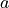of a voxel located at coordinates (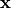) within the fixed image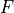is given by: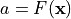and similarly, the intensity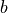of a voxel at coordinates (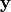) within the moving image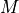is given by: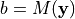We want to apply a coordinate transform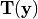to the moving image such that it registers best with the fixed image. However, sinceandcould have been obtained from different imaging modalities, we cannot simply minimize the error between intensitiesand. Instead, we must attempt to maximize the amount of information shared between the two images. The statistical Mutual Information can therefore be used as a similarity metric:

(1)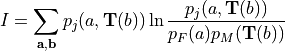which depends on the probability distributions of the voxel intensities for the static and moving images. We now viewandas random variables with associated probability distribution functions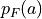and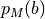and joint probability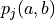. Note that applying the spatial transformation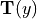towill result in modification of. This effect is implied with the notation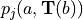. Furthermore, if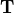results in voxels being displaced outside of the image,will change. Perhaps more importantly, ifresults in a voxel being remapped to a location that falls between points on the voxel grid, some form of interpolation must be employed to obtain, which will modify. These effects are implied with the notation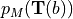.

The method of interpolation used to obtaingivenis important both in terms of execution speed and solution convergence. Here we will discuss the Partial Volume Interpolation method proposed by Maes et. al.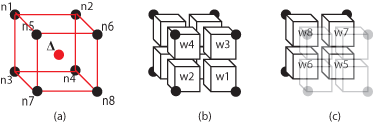Figure 1(a) depicts the situation where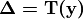has displaced a voxel (red) to the center of a neighborhood of 8 other voxels (black). The interpolation method divides the volume defined by the 8-nearest neighbors (red) into 8 partial volumes (w1 - w8) that all share the red voxel’s location as a common vertex Figure 1(b-c). We can more formally define the 8 partial volumes as a function of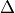:

(2)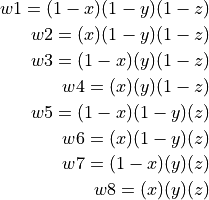Noting thatOnce the partial volumes have been computed, they are placed into the histograms of their corresponding voxels in the 8-neighborhood. Partial volume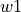is placed into the histogram bin associated with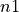,with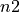, etc. This method is used in computing bothand.

We now have a complete expression for the mutual information similarity metric, which we will use as a cost function that describes the quality of the registration.

(3)We can attempt to achieve the optimal registration by maximizing this cost function, which is achieved through modification of the coordinate transformationapplied to the moving image. It is important to note that thecould be any type of conceivable coordinate transformation. For example,could represent a set of affine transforms to be applied to the moving image. Here, however, we will be focusing on a parametrically defined nonrigid coordinate transformation. The transformationis characterized by the displacement field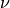, which defines how individual voxels within the moving imageshould be displaced such that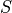andare optimally registered. This displacement field is computed at every voxel given the B-spline coefficients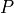defined for the sparse array of control-points:

(4)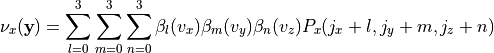where (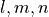) form the indices of the 64 control points in the immediate vicinity of a voxel located at (). Note, () forms the voxel’s offsets within the region bound by the 8 control-points in the immediate vicinity of the voxel. For simplicity, we refer to such a region as a tile.

Having defined the coordinate transformationin terms of the B-spline coefficients, it is becomes apparent that the mutual information may be maximized through optimization of. If this is to be accomplished via the method of gradient descent, an analytic expression for the gradientmust be derived. The chain rule is employed to separate the expression into two partial derivatives with the first being dependent on the similarity metric employed and the second on the type of transform employed:

(5)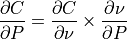where the second term is easily obtained by taking the derivative of (4) with respect to: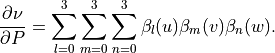Referring again to the first term of (5), it is important to realize that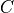and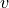are coupled through the probability distribution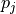and are therefore directly affected by the 8 neighborhood partial volume interpolation. This becomes further apparent when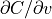is further decomposed: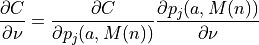(6)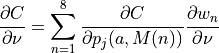where the first term may be found by taking the derivative of (3) with respect to the joint distribution:

(7)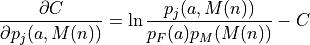see {A}.

The second term,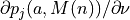, describes how the joint distribution changes with the vector field. Explicitly stated, a displacement vector locally transforms the coordinates of a voxel in the moving imagesuch that: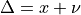From Figure fig:pv8 it becomes immediately apparent that as the vector field is modified the partial volumes-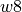to be inserted into the moving and joint histograms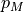andwill change in size. Consequently,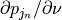can be found for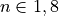by taking the derivative of (2) with respect to each of the Cartesian directions.

Therefore, as prescribed by (6), computingat a given voxel ininvolves first cycling through the 8-bins corresponding to the 8 nearest neighbors used during the partial volume interpolation and computing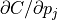for each, which is scalar and therefore the same for all Cartesian directions. The 8values are each weighted by one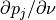. Finally, once this process has been completed for all 8 bins, the resultingvalues is assimilated into the sparse coefficient arrayby way of (5). Once this is completed for all voxels, the next optimizer iteration begins and the process is repeated with a modified coefficient array.

NOTE

It is important to note that if all 8 histograms associated with the neighborhood are equal, the value of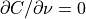. This is a direct result of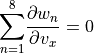and similarly for the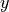- and-directions. However, when the 8 histograms within the neighborhood are not equal, this will result in the gradientbeing “pulled” in the Cartesian direction of voxels associated with large, which is desirable when attempting to maximize MI.